SELF 10 S.B. Karavashkin and O.N. Karavashkina5. Infinite gravity compression We showed relativistic constructions to be fully abstracted from the physical meaning of processes; we can see a next bright example in the problem of dying stars. The paper by Oppenheimer and Snyder  whose name we have put to the title of this item is one of typical in this sense. In this paper the authors study "stars of large masses (> 0,7 M)  that have burned out their nuclear sources of energy. Such star collapses under affection of its own gravity field, releasing the energy … If initially the star mass was enough small, or if a sufficient part of mass can be blown out from the surface of star by its emission (? - Authors), or immediately taken away as the radiation (? - Authors), or if the momentum of star was quite large it to be separated into small fragments (? - Authors), the rest of mass can form a stable static distribution - white dwarf. We will consider the case when this does not occur" [28, p. 353- 354]. We from our side also will not analyse a clearly artificial and unfounded reasons listed by the authors, which could bring the change of initial mass of star. We will focus our attention on the methods to prove the ability of celestial bodies to collapse. Unfortunately, the methods are same. To begin, Oppenheimer without 'excessive' pondering introduces the Schwarzschild's metric, which is known to be describing the field of point gravitating mass - and, as we showed above, describing erroneously. But the main, this metric describes the field out of mass, and Oppenheimer showed his understanding of this shade when introduced the metric: "If in this case we may at the later stages of compression neglect the gravity action of any radiation either substance leaving the star, as well as the deviations from spherical symmetry caused by rotation, then out of the limits rb of distribution of stellar substance (italicised by us - Authors) the interval has to take the form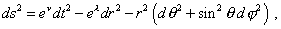(53)
 where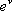= 1- r0 /r  and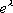= (1- r0 / r)-1 . Here  r0 is the stellar gravity radius connected with its gravity mass m by the equality r0 = 2mg / c2 , it is constant" [28, p. 354]. Naturally, such statement of problem does not satisfy the authors, even though they distorted the definition of Schwarzschild's radius. As we revealed before, this means the singularity of metric, not the radius of gravitating body, which in Schwarzschild's problem was infinitesimal. So Oppenheimer and Snyder immediately apply the relativistic method to 'adjust' the phenomenology. At the first stage the authors introduce: "As the pressure of stellar substance is insufficient to counteract the forces of own gravity attraction of star, it probably will begin compressing, so that the boundary rb will unavoidably diminish to the gravity radius r0 . The local observer located nearby the stellar surface, where the pressure anyway has to be low, will see that the substance goes to the depths with the speed very close to that of light. But a far observer will see this motion in (1- r0 / r)-1 times slower" [ibidem]. It is easy to understand from the cited statement of the problem that the authors have crudely violated both classical and relativistic idea of the phenomenology of real processes. This does not advance the authors to the result they want to achieve, as the conditions for which Schwarzschild's metric (53) has been written remain inviolable. For the relativists, this inconvenience means the only: they need to retain the metric, changing the conditions, - and the authors start it. "Let us think the substance inside the star to be distributed in spherical symmetry. Then we may take the interval as (53)" [ibidem]. As we see, this is very simple. If no substance - the metric (53) is true. If the substance is present - too, if it is distributed in spherical symmetry. No difference. With it the tensor of energy-momentum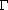consists already of two parts: "1) the contribution of substance corresponding to electrons, protons, neutrons and nuclei, and 2) the contribution of radiation" [ibidem, p. 355]. And "we can think the contribution of substance as a tensor for a liquid moving with the radius, for which in the accompanying coordinates a definite relation between the pressure, density and temperature is true" [ibidem, p. 355]. Thus, the tensor is similar to that for liquid, but for such liquid whose surface is shifted to the centre with the speed close to that of light. If this is so, as we revealed above, for a liquid, (47) will provide the relationship in volume variation and counter-pressure, and it is easily to calculate that with outer pressure, which the substance is able to produce in a body with the mass comparable with that of Sun, so high rate of substance compression basically cannot be true, at least because with such mass the substance basically cannot be compressed without counteraction from inside. Next, in order to accelerate a body (the more its parts) to the speed close to that of light, in the field of gravitating body with the mass close to that of Sun, it needs the distance immensely larger than the size of the very gravitating body. So the substance at the body boundary, with all clearest wish of the authors, cannot 'go to the depths with the speed very close to that of light'. Furthermore, as we showed in the previous item, the light deformation in celestial bodies having the size like Sun is not so large, the body to be unable to resist the compression; this means, in the body there will arise an effective counteraction that will compensate the striving of substance to penetrate deeper. In the view of Relativity, Oppenheimer and Snyder also are highly mistaken. For two observers, if one of them is near the body's surface and another is distanced from the surface, the speed of substance flow on the surface of collapsing body also will not seem to be so much different as the authors predict. As we revealed in the item 2, it follows from Einstein's views that for observers located at the inertial reference frame in gravitating field, the light velocity is constant, as namely this value Einstein puts to all his formulas. The fact that later he denies his statement of problem is already insufficient from the view of physics, as the formulas have been yielded namely under stipulation of constant speed of light in the view of inertial observer. Naturally, with so deep inconsistency in the reality and the authors' wish to stipulate the collapse of the system, they could not put their understanding in agreement even in frames of clearly unphysical representation of Schwarzschild's metric. And they confessed: "We did not succeed to integrate these equations (the field equations - Authors) doing not putting the pressure equal to zero" [28, p. 355]. But this did not stop a least Oppenheimer and Snyder in their striving to prove the collapse anyway. They again and again modify the conditions of problem. In the end of ends, all versions were reduced to those which appeared convenient to achieve the target, but are inconvenient by virtue of conditions inconsistent with the collapsing star at which they consider the current problem: "To answer this question, we will find the solution of field equations for the limiting case of tensor of energy-momentum, when the pressure is zero (! - Authors). In absence of pressure, the field equations do not have statical solutions, except when all components ofvanish. Setting p = 0 , we come to a free gravitation collapse of substance". And with it, "as we consider the accompanying coordinates and the pressure is zero,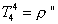(54)
 [ibidem, p. 356- 357]. Thus, it follows from the modified statement of problem that in the model of star, the pressure in the star has to be zero, tensor of energy-momentum also has to be zero (any processes are absent and the star has no energy), but the density has to be non-zero and in some way uniformly distributed in the star. The only question is, what concern this so-to-call model can have to real processes? Nothing to say of instabilities, redistribution of mass, mutual affection of substance in the star, of thermodynamical processes; but even in limits of relativistic geometrical approach? Of course, it is irrelevant. But namely these solutions separated from the stipulations under which they were derived have been elevated by relativists to the level of unquestionable, thoroughly substantiated, and even observed by some people somewhere.

Contents: / 1 / 2 / 3 / 4 / 5 / 6 / 7 / 8 / 9 / 10 / 11 / 12 / 13 / 14 / 15 / 16 / 17 /